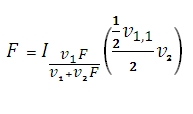# SQL Server F.INV function

Updated: 9 August 2010

Use FINV to calculate the inverse of the F probability distribution. A frequent use of the F-distribution is to test whether two observed samples have the same variance. The F-distribution cumulative distribution function (cdf) is given in terms of the incomplete beta function:Syntax
SELECT [wctStatistics].[wct].[FINV] (
<@Probability, float,>
,<@Degrees_freedom1, float,>
,<@Degrees_freedom2, float,>)
Arguments
@Probability
is a probability associated with the F-distribution cdf. @Probability is an expression of type float or of a type that can be implicitly converted to float
@Degrees_freedom1
is the numerator degrees of freedom. @Degrees_freedom1 is an expression of type float or of a type that can be implicitly converted to float
@Degrees_freedom2
is the denominator degrees of freedom. @Degrees_freedom2 is an expression of type float or of a type that can be implicitly converted to float
Return Types
float
Remarks
·         If @Probability < 0 or @Probability > 1, FINV returns an error.
·         @Degrees_freedom1 and @Degrees_freedom2  are truncated to zero decimal places.
·         If @Degrees_freedom1 < 1 or @Degrees_freedom1 > 10^10, FINV returns an error.
·         If @Degrees_freedom2 < 1 or @Degrees_freedom2 > 10^10, FINV returns an error.
·         FINV uses root-finding algorithms and iteration to satisfy the condition that
FDIST(FINV(@Probability,@Degrees_freedom1, @Degrees_freedom2), @Degrees_freedom1, @Degrees_freedom2)-@Probability = 0 to eight decimal places.
Examples

Select
wct.FINV(.05, 6, 10)

This produces the following result
----------------------
3.21717454739921

(1 row(s) affected)

Select
round(wct.FDIST(wct.FINV(.05, 6, 10), 6, 10)-.05, 8)

This produces the following result
----------------------
0

(1 row(s) affected)

### SupportCopyright 2008-2023 Westclintech LLC         Privacy Policy        Terms of Service